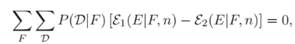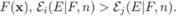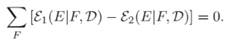# Prove Part 1 of Theorem 9.1, i.e., that uniformly averaged over all target functions F, E1(E|F, n) − E2(E|F, n) = 0. Summarize and interpret this result in words. Part 1 Theorem 9.1 says that uniformly averaged over all target functions the expected error for all learning algorithms is the same, i.e., for any two learning algorithms. In short, no matter how clever we are at choosing a “good” learning algorithm P1(h|D), and a “bad” algorithm P2(h|D) (perhaps even random guessing, or a constant output), if all target functions are equally likely, then the “good” algorithm will not outperform the “bad” one. Stated more generally, there are no i and j such that for all  Furthermore, no matter what algorithm you use, there is at least one target function for which random guessing is a better algorithm. Assuming the training set can be learned by all algorithms in question, then Part 2 states that even if we know D, then averaged over all target functions no learning algorithm yields an off-training set error error that is superior to any other, i.e., Parts 3 & 4 concern non-uniform target function distributions, and have related in- terpretations (Problems 2 – 5). Example 1 provides an elementary illustration.

Question
1 views

Prove Part 1 of Theorem 9.1, i.e., that uniformly averaged over all target functions F, E1(E|F, n) − E2(E|F, n) = 0. Summarize and interpret this result in words.

Part 1 Theorem 9.1

says that uniformly averaged over all target functions the expected error

for all learning algorithms is the same, i.e.,for any two learning algorithms. In short, no matter how clever we are at choosing

a “good” learning algorithm P1(h|D), and a “bad” algorithm P2(h|D) (perhaps even

random guessing, or a constant output), if all target functions are equally likely, then

the “good” algorithm will not outperform the “bad” one. Stated more generally,

there are no i and j such that for allFurthermore, no

matter what algorithm you use, there is at least one target function for which random

guessing is a better algorithm.

Assuming the training set can be learned by all algorithms in question, then Part 2

states that even if we know D, then averaged over all target functions no learning

algorithm yields an off-training set error error that is superior to any other, i.e.,Parts 3 & 4 concern non-uniform target function distributions, and have related in-

terpretations (Problems 2 – 5). Example 1 provides an elementary illustration.

### This question hasn't been answered yet.